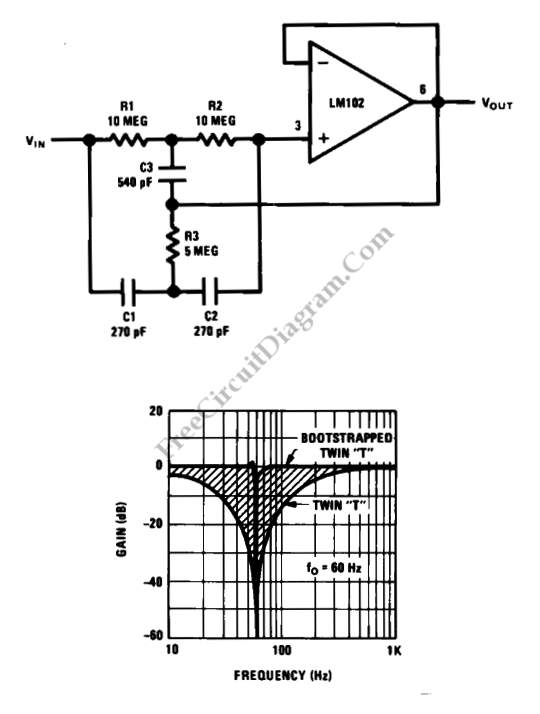# Bootstrapped Twin-T Notch Filter Produces High QThis is a Bootstrapped Twin-T Notch Filter circuit. This circuit can generate high Q by combining the twin “T” with LM102 voltage follower. The generated Q is greater than 50. This circuit uses small capacitors since the LM102 uses the large resistance values in the “T”. Beside that, voltage follower also used as buffer that gives high input resistance of the LM102 and low output resistance. This circuit can be used at high frequencies, since the fast response of the follower. When the follower added, the frequency and depth of notch will not changed. Here is the schematic diagram of the circuit:The lower figure is the response of a normal twin “T” and the response with the follower added. This circuit is called bootstrapped Twin-T Notch Filter because the junction of C3 and R3 are connected to ground. So that junction is bootstrapped to the follower output. This circuit increases the Q in propotion to the signal feedback value to C3 and R3. The frequency and depth of notch because of the very low output impedance of follower. [Circuit’s schematic diagram source: National Semiconductor Application Note]